×

Search anything:

# time command in Linux

#### Linux Software EngineeringIndian Technical Authorship Contest starts on 1st July 2023. Stay tuned.

When executing commands in Linux, we may want to know how long it takes or how much of the system resources the command uses. In such cases, we use the time command that comes before the command to be executed. We also use this command to estimate the run time of algorithms for optimization purposes.

1. Introduction.
2. Introduction.
3. Syntax.
4. Usage.
5. Summary.
6. References.

## Introduction.

In Linux, to use the time command, we can either provide the whole path as we shall see shortly, or use the time command which is common or precede the time command with a backslash. This is because there exist three versions, to check the current version we are using we execute the command:

``````\$ type time
``````

For BASH we expect:

``````time is a shell keyword
``````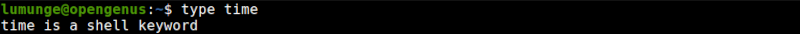For ZSH we expect:

``````time is a reserved keyword
``````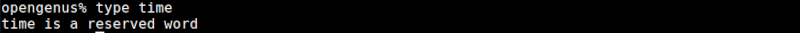For GNU(sh) we expect:

``````time is /usr/bin/time
``````

## Syntax.

The time command is written before a command we are executing.
For example to time the execution of a command we write:

``````\$ time [COMMAD]
``````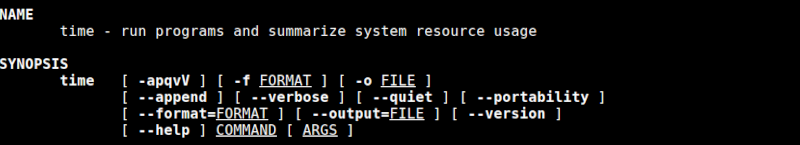On most applications of this command, we will find that we don't need command options however, some options come in handy such as:

• -o, to send the command output to a file.
• -v to display a verbose output.
• -p, to display output in POSIX format.

## Usage.

To time the execution of a command in the Linux terminal, all we need to do is precede the command with time. For example to know the execution time of a curl/wget command that fetches a remote resource we write:

``````\$ time curl -s www.google.com > index.html
``````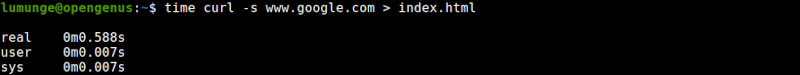From the output we have three rows with two columns;

• real represents the actual time it took the command to execute.
• user is the amount of time the processor spent in user mode.
• sys is the time the processor spent in kernel mode.

In user mode, a process can access hardware or reference memory outside of its allocation. For access to such resources, this process sends queries to the kernel and if the kernel approves, this process enters the kernel mode of execution until the requirement is satisfied after which it switches back to user mode execution.

To print output in portable POSIX format we use the -p option as follows:

``````\$ time -p curl -s www.google.com > index.html
``````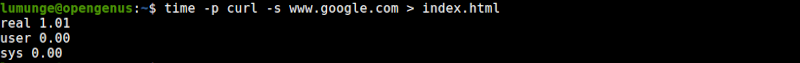We can also send the time command output to a file by using the -o option followed by the file we want to send output to. For example, to send output to a file out.txt, we write:

``````\$ /usr/bin/time -o timeFile.txt curl -s www.google.com > index.html
``````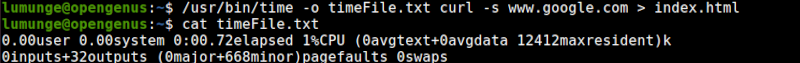In this case, we use the path to the time command binary since the built-in command won't support the -o option.
We get this path by executing \$ which time.

To get output in verbose, we use the -v option as follows:

``````\$ /usr/bin/time -v curl -s www.google.com > index.html
``````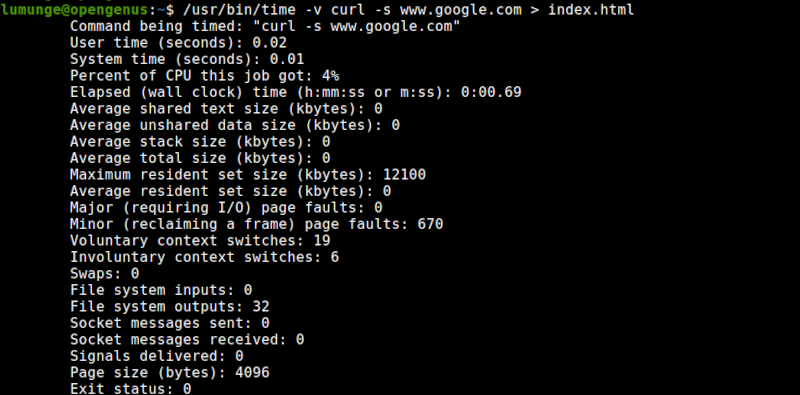We can also opt to format the command output. The default format is h:m:s, that is hours, minutes, and seconds:

``````\$ /usr/bin/time -f "\t%C [Command name],\t%E [Time Elapsed]"  curl -s www.google.com > index.html
``````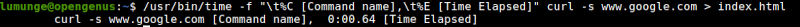Notice how we have used \t, this is for spacing the output using tabs. The %C will print out the command name, %E prints out the time elapsed by this command.

For more on formatting we can read the manual page of this command by executing the command:

``````\$ man time
``````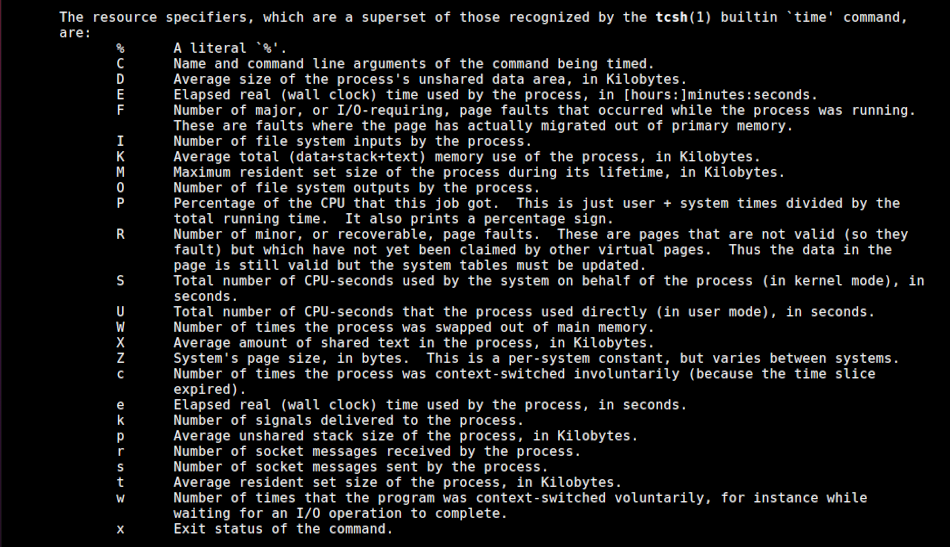Apart from Linux commands and the command line, we can also use it in programs to time the execution and improve the performance of code. For example, we have the following algorithm to find the nth Fibonacci number.
naive.c:

``````#include<stdio.h>
#include<stdlib.h>

int fib(int n){
if(n <= 1) return n;
return fib(n-1) + fib(n-2);
}
int main(int argc, char *argv[]){
int n = atoi(argv);
printf("%d \n", fib(n));

return 0;
}
``````

The above is a naive implementation of the algorithm that consumes alot of system resources for large inputs. We time this and compare it with the following optimal algorithm.

Now to optimize the algorithm using dynamic programming to improve run time:
optimal.c

``````#include<stdio.h>
#include<stdlib.h>

int fib(int n){
int dp[n+2];
dp = 0; dp = 1;
for(int i = 2; i <= n; i++)
dp[i] = dp[i-1] + dp[i-2];

return dp[n];
}
int main(int argc, char *argv[]){
int n = atoi(argv);
printf("%d \n", fib(n));
return 0;
}
``````

Here is the execution times for finding the 40th Fibonacci number using both algorithms: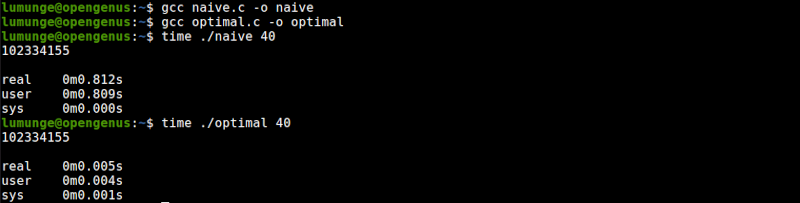Notice the differences in run times of the two algorithms?

## Summary.

The time command is used to time a command in Linux. Apart from timing the execution of Linux commands, we also use it to determine the run time of an algorithm for optimization purposes. It can also give us information on how a command or algorithm uses system resources such as memory, the stack, and much more.

#### Erick Lumunge

Erick is a passionate programmer with a computer science background who loves to learn about and use code to impact lives positively.